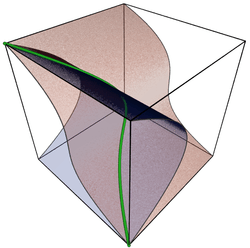# Twisted cubic

In mathematics, a twisted cubic is a smooth, rational curve C of degree three in projective 3-space P3. It is a fundamental example of a skew curve. It is essentially unique, up to projective transformation (the twisted cubic, therefore). It is generally considered to be the simplest example of a projective variety that is not linear or a hypersurface, and is given as such in most textbooks on algebraic geometry. It is the three-dimensional case of the rational normal curve, and is the image of a Veronese map of degree three on the projective line.

## DefinitionIt is most easily given parametrically as the image of the mapwhich assigns to the homogeneous coordinatethe valueIn one coordinate patch of projective space, the map is simply the moment curveThat is, it is the closure by a single point at infinity of the affine curve.

Equivalently, it is a projective variety, defined as the zero locus of three smooth quadrics. Given the homogeneous coordinates [X:Y:Z:W] on P3, it is the zero locus of the three homogeneous polynomialsIt may be checked that these three quadratic forms vanish identically when using the explicit parameterization above; that is, substituting x3 for X, and so on.

In fact, the homogeneous ideal of the twisted cubic C is generated by three algebraic forms of degree two on P3. The generators of the ideal are## Properties

The twisted cubic has an assortment of elementary properties:

• It is the set-theoretic complete intersection of XZ-Y2 and, but not a scheme-theoretic or ideal-theoretic complete intersection (the resulting ideal is not radical, sinceis in it, butis not).
• Any four points on C span P3.
• Given six points in P3 with no four coplanar, there is a unique twisted cubic passing through them.
• The union of the tangent and secant lines, the secant variety, of a twisted cubic C fill up P3 and the lines are pairwise disjoint, except at points of the curve itself. In fact, the union of the tangent and secant lines of any non-planar smooth algebraic curve is three-dimensional. Further, any smooth algebraic variety with the property that every length four subscheme spans P3 has the property that the tangent and secant lines are pairwise disjoint, except at points of the variety itself.
• The projection of C onto a plane from a point on a tangent line of C yields a cuspidal cubic.
• The projection from a point on a secant line of C yields a nodal cubic.
• The projection from a point on C yields a conic section.

## References

• Harris, Joe (1992), Algebraic Geometry, A First Course, New York: Springer-Verlag, ISBN 0-387-97716-3.